# Python Matplotlib Tutorial: Plotting Data And Customisation

Matplotlib is a popular Python library that can be used to create data visualizations quite easily. It is probably the single most used Python package for 2D-graphics along with limited support for 3D-graphics. It provides both, a very quick way to visualize data from Python and publication-quality figures in many formats. Also, It was designed from the beginning to serve two purposes:

1. Allow for interactive, cross-platform control of figures and plots
2. Make it easy to produce static vector graphics files without the need for any GUIs.

Much like Python itself, Matplotlib gives the developer complete control over the appearance of their plots. It tries to make easy things easy and hard things possible. We can generate plots, histograms, power spectra, bar charts, error charts, scatter plots, etc. with just a few lines of code. For simple plotting, the pyplot module within the matplotlib package provides a MATLAB-like interface to the underlying object-oriented plotting library. It implicitly and automatically creates figures and axes to achieve the desired plot.In this Python Matplotlib tutorial, we will cover the following topics:

## How to import Python Matplotlib

To get started with Python Matplotlib, we first import the package. It is a common practice to import matplotlib.pyplot using the alias as plt. The pyplot being the sub-package within Matplotlib provides the common charting functionality. Also, if we are working in a Jupyter Notebook, the line %matplotlib inline becomes important, as it makes sure that the plots are embedded inside the notebook. This is demonstrated in the example below:

import matplotlib.pyplot as plt

%matplotlib inline


Matplotlib do not fall under the Python Standard Library and hence, like any other third-party library, it needs to be installed before it can be used. It can be installed using the command pip install matplotlib.

You can go through the following blog to understand how to install and import Python packages. Thus, you have understood how to import matplotlib. Before we start plotting graphs, let us first understand the key terms in the next section of the Python matplotlib tutorial.

## Basic Terms and Concepts in Matplotlib

Python Matplotlib allows creating a wide variety of plots and graphs. Matplotlib is a large project and can seem daunting at first. However, we will start learning the components and it should feel much smaller and approachable.

Different sources use 'plot' to mean different things. So let us begin by defining specific terminology used across the domain.

• Figure is the top-level container in the hierarchy. It is the overall window where everything is drawn. We can have multiple independent figures, and each figure can have multiple Axes. It can be created using the figure method of pyplot module.

• Axes is where the plotting occurs. The axes are effectively the area that we plot data on. Each Axes has an X-Axis and a Y-Axis.

The example mentioned below illustrates the use of the above-mentioned terms:

fig = plt.figure()
<Figure size 432x288 with 0 Axes>


Upon running the above example, nothing happens really. It only creates a figure of size 432 x 288 with 0 Axes. Also, Python Matplotlib will not show anything until told to do so. Python will wait for a call to show method to display the plot. This is because we might want to add some extra features to the plot before displaying it, such as title and label customization. Hence, we need to call plt.show() method to show the figure as shown below:

plt.show()


As there is nothing to plot, there will be no output. While we are on the topic, we can control the size of the figure through the figsize argument, which expects a tuple of (width, height) in inches.

fig = plt.figure(figsize=(8, 4))
<Figure size 576x288 with 0 Axes>
plt.show()


### Axes

All plotting is done with respect to an Axes. An Axes is made up of Axis objects and many other things. An Axes object must belong to a Figure. Most commands that we will ever issue in Python mathplotlib will be with respect to this Axes object. Typically, we will set up a Figure, and then add Axes on to it. We can use fig.add_axes but in most cases, we find that adding a subplot fits our need perfectly. A subplot is an axes on a grid system.

• add_subplot method adds an Axes to the figure as part of a subplot arrangement.
""" Example 1 """
# Creating figure
fig = plt.figure()

# Creating subplot
ax = fig.add_subplot(111) # Subplot with 1 row and 1 column at the index 1
plt.show()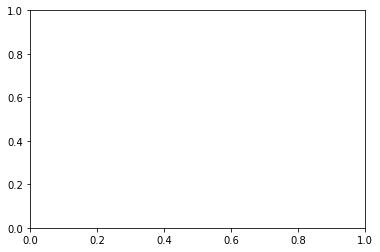The above code adds a single plot to the figure fig with the help of add_subplot() method. The output we get is a blank plot with axes ranging from 0 to 1 as shown above. In Python matplotlib, we can customize the plot using a few more built-in methods. Let us add the title, X-axis label, Y-axis label, and set limit range on both axes. This is illustrated in the below code snippet.

""" Example 2 """
fig = plt.figure()

# Creating subplot/axes

# Setting axes/plot title
ax.set_title('An Axes Title')

# Setting X-axis and Y-axis limits
ax.set_xlim([0.5, 4.5])
ax.set_ylim([-3, 7])

# Setting X-axis and Y-axis labels
ax.set_ylabel('Y-Axis Label')
ax.set_xlabel('X-Axis Label')

# Showing the plot
plt.show()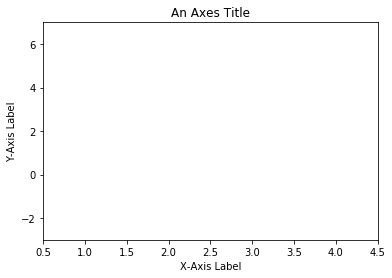Python Matplotlib's objects typically have lots of explicit setters, i.e. methods that start with set_<something> and control a particular option. Setting each option using explicit setters becomes repetitive, and hence we can set all required parameters directly on the axes using the set method as illustrated below:

""" Example 2 using the set method """
fig = plt.figure()

# Creating subplot/axes

# Setting title and axes properties
ax.set(title='An Axes Title', xlim=[0.5, 4.5], ylim=[-3, 7], ylabel='Y-Axis Label', xlabel='X-Axis Label')

plt.show()


The set method does not apply to Axes; it applies to more-or-less all Python matplotlib objects.

The above code snippet the same output as figure 2 above using the set method will all required parameters passed as arguments to it.

### Axes method v/s pyplot

Interestingly, almost all methods of axes objects in Python Matplotlib exist as a method in the pyplot module. For example, we can call plt.xlable('X-Axis Label') to set the label of X-axis (plt being an alias for pyplot), which in turn calls ax.set_xlabel('X-Axis Label') on whichever axes is current.

""" Example 3 """
# Creating subplots, setting title and axes labels using pyplot
plt.subplots()
plt.title('Plot using pyplot')
plt.xlabel('X-Axis Label')
plt.ylabel('Y-Axis Label')
plt.show()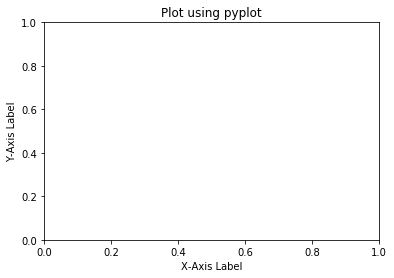The code above is a bit easier and has fewer variables to construct a plot. It uses implicit calls to axes method for plotting. However, if we take a look at "The Zen of Python" (try import this), it says:

"Explicit is better than implicit."

While very simple plots, with short scripts, would benefit from the conciseness of the pyplot implicit approach, when doing more complicated plots, or working within larger scripts, we will want to explicitly pass around the axes and/or figure object to operate upon. We will be using both approaches in this Python matplotlib tutorial, wherever it deems appropriate.

Anytime we see something like below:

fig = plt.figure()


can be replaced with the following:

fig, ax = plt.subplots()


Both versions of code produce the same output. However, the latter version is cleaner.

### Multiple Axes

A figure can have more than one Axes on it. In Python matplotlib, the easiest way is to use plt.subplots() call to create a figure and add the axes to it automatically. Axes will be on a regular grid system. For example,

""" Example 4 """
# Creating subplots with 2 rows and 2 columns
fig, axes = plt.subplots(nrows=2, ncols=2)
plt.show()


Upon running the above code, Python Matplotlib would generate a figure with four subplots added arranged in two rows and two columns as shown below: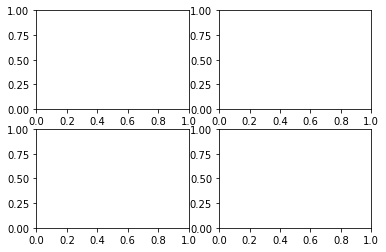The axes object that was returned here would be a 2D-NumPy array, and each item in the array is one of the subplots. Therefore, when we want to work with one of these axes, we can index it and use that item's methods. Let us add the title to each subplot using the axes methods.

""" Example 5 """
# Create a figure with four subplots and shared axes
fig, axes = plt.subplots(nrows=2, ncols=2, sharex=True, sharey=True)
axes[0, 0].set(title='Upper Left')
axes[0, 1].set(title='Upper Right')
axes[1, 0].set(title='Lower Left')
axes[1, 1].set(title='Lower Right')
plt.show()


The above code created a figure with four subplots and shared X and Y axes. Axes are shared among subplots in row-wise and column-wise manner. We then set a title to each subplot using the set method for each subplot. Subplots are arranged in a clockwise fashion with each subplot having a unique index. The output is shown below: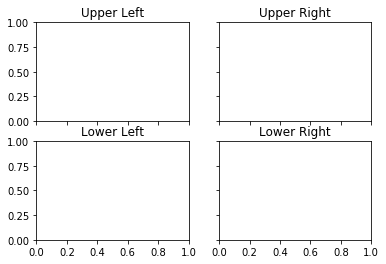## Plotting Data in Python Matplotlib

So far in this Python matplotlib tutorial, we have discussed a lot about laying things out, but we haven't really discussed anything about plotting data yet. Python Matplotlib has various plotting functions. Many more than we will discuss and cover here. However, a full list or gallery1 can be a bit overwhelming at first. Hence, we will condense it down and attempt to start with simpler plotting and then move towards more complex plotting. The plot method of pyplot is one of the most widely used methods in Python Matplotlib to plot the data. The syntax to call the plot method is shown below:

plot([x], y, [fmt], data=None, **kwargs)


The coordinates of the points or line nodes are given by x and y. The optional parameter fmt is a convenient way of defining basic formatting like color, market, and style. The plot method is used to plot almost any kind of data in Python. It tells Python what to plot and how to plot it, and also allows customization of the plot being generated such as color, type, etc.

### Line Plot

In Python matplotlib, a line plot can be plotted using the plot method. It plots Y versus X as lines and/or markers. Below we discuss a few scenarios for plotting line. To plot a line, we provide coordinates to be plotted along X and Y axes separately as shown in the below code snippet.

""" Example 6 """
# Defining coordinates to be plotted on X and Y axes respectively
x = [1.3, 2.9, 3.1, 4.7, 5.6, 6.5, 7.4, 8.8, 9.2, 10]
y = [95, 42, 69, 11, 49, 32, 74, 62, 25, 32]

# Plot lists 'x' and 'y'
plt.plot(x, y)

# Plot axes labels and show the plot
plt.xlabel('X-axis Label')
plt.ylabel('Y-axis Label')
plt.show()


The above code plots values in the list x along the X-axis and values in the list y along the Y-axis. The output is shown below: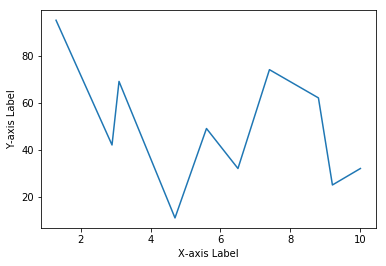The call to plot takes minimal arguments possible, i.e. values for Y-axis only. In such a case, Python Matplotlib will implicitly consider the index of elements in list y as the input to the X-axis as demonstrated in the below example:

""" Example 7 """
# Defining 'y' coordinates
y = [95, 42, 69, 11, 49, 32, 74, 62, 25, 32]

# Plot list 'y'
plt.plot(y)

# Plot axes labels and show the plot
plt.xlabel('Index Values')
plt.ylabel('Elements in List Y')
plt.show()


Here, we define a list called y that contains values to be plotted on Y-axis. The output is shown below: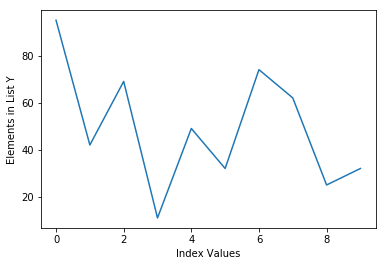The plots created above uses the default line style and color. The optional parameter fmt in the plot method is a convenient way for defining basic formatting like color, marker, and line-style. It is a shortcut string notation consisting of color, marker, and line:

fmt = '[color][marker][line]'

Each of them is optional. If not provided, the value from the style cycle is used. We use this notation in the below example to change the line color:

""" Example 8 """
# Plot line with green color
plt.plot(y, 'g')

# Plot axes labels and show the plot
plt.xlabel('Index Values')
plt.ylabel('Elements in List Y')
plt.show()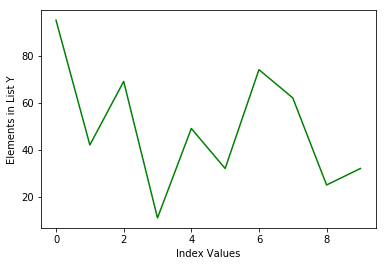Following the fmt string notation, we changed the color of a line to green using the character g which refers to the line color. Likewise, markers are added using the same notation as shown below:

""" Example 9 """
# Plot continuous green line with circle markers
plt.plot(y, 'go-')

# Plot axes labels and show the plot
plt.xlabel('Index Values')
plt.ylabel('Elements in List Y')
plt.show()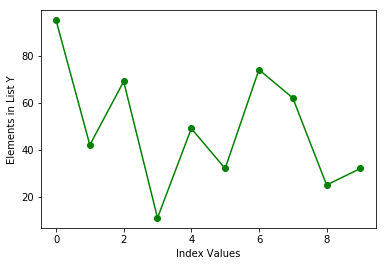Here, the fmt parameters g refers to the green color, o refers to circle markers and - refers to a continuous line to be plotted. This formatting technique allows us to format a line plot in virtually any way we like. It is possible to change marker style by tweaking marker parameter in the fmt string as shown below:

""" Example 10 """
# Plot continuous green line with circle markers
plt.plot(y, 'g*-')

# Plot axes labels and show the plot
plt.xlabel('Index Values')
plt.ylabel('Elements in List Y')
plt.show()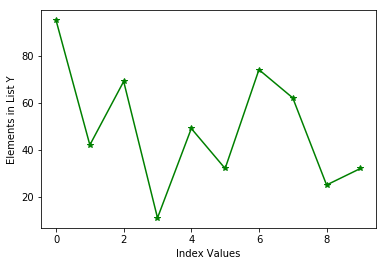In the above plots, the line and markers share the same color, i.e. green specified by the fmt string. If we are to plot line and markers with different colors, we can use multiple plot methods to achieve the same.

""" Example 11 """
# Plot list 'y'
plt.plot(y, 'g')

# Plot red circle markers
plt.plot(y, 'ro')

# Plot axes labels and show the plot
plt.xlabel('Index Values')
plt.ylabel('Elements in List Y')
plt.show()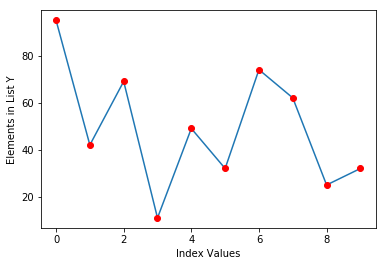The above code plots line along with red circle markers. Here, we first plot the line with the default style and then attempt to plot markers with attributes r referring to red color and o referring to circle. On the same lines, we can plot multiple sets of data using the same technique. The example given below plots two lists on the same plot.

""" Example 12 - Technique 1"""
# Define two lists
y = [95, 42, 69, 11, 49, 32, 74, 62, 25, 32]
y2 = [35, 52, 96, 77, 36, 66, 50, 12, 35, 63]

# Plot lists and show them
plt.plot(y, 'go-')
plt.plot(y2, 'b*--')

# Plot axes labels and show the plot
plt.xlabel('Index Values')
plt.ylabel('Elements in Lists')
plt.show()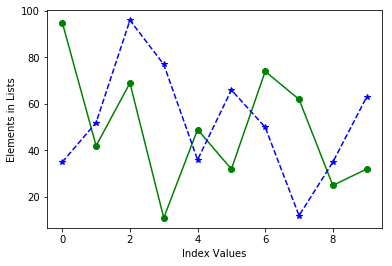We can achieve the same result as shown above using the different technique as shown below:

""" Example 12 - Technique 2"""
# Plot lists and show them
plt.plot(y, 'go-', y2, 'b*--')

# Plot axes labels and show the plot
plt.xlabel('Index Values')
plt.ylabel('Elements in Lists')
plt.show()


Essentially, the plot method makes it very easy to plot sequential data structure such as list, NumPy arrays, pandas series, etc. Similar to plotting lists, we can plot NumPy arrays directly via the plot method. Let us plot NumPy one dimensional array. As we are executing codes directly in IPython console, calling the plt.show() is not required and hence, we will not be calling the same in subsequent examples. However, remember, it is absolutely necessary to call it while writing Python code to show a plot.

""" Example 13 """
# Importing NumPy library
import numpy as np

# Drawing 30 samples from a standard normal distribution into an array 'arr'
arr = np.random.normal(size=30)

# Plotting 'arr' with dashed line-style and * markers
plt.plot(arr, color='teal', marker='*', linestyle='dashed')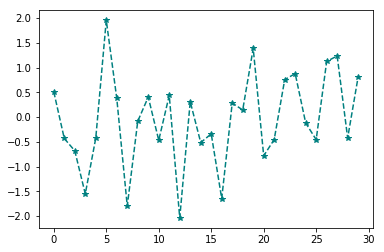In the above example, we draw thirty samples from a normal distribution into an array arr which in turn gets plotted in dashed line along with asterisk markers. Plotting two-dimensional arrays follows the same pattern. We provide a 2-D array to a plot method to plot it.

""" Example 14 """
# Creating a two dimensional array 'arr_2d' with 40 samples and shape of (20, 2)
arr_2d = np.random.normal(size=40).reshape(20, 2)

# Plotting the array
plt.plot(arr_2d)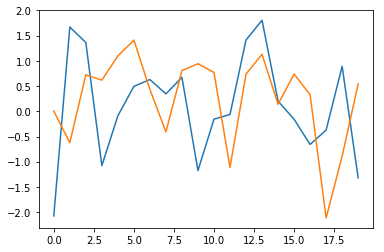Let us now move our focus to plot pandas data structures. The pandas library use the standard convention as Python matplotlib for plotting directly from its data structures. The pandas also provide a plot method which is equivalent to the one provided by Python matplotlib. Hence, the plot method can be called directly from pandas Series and DataFrame objects. The plot method on Series and DataFrame is just a simple wrapper around plt.plot(). The below example illustrates plotting pandas Series object:

""" Figure 15 """
# Importing necessary libraries
import pandas as pd
import numpy as np

# Creating pandas Series with 50 samples drawn from normal distribution
ts = pd.Series(np.random.normal(size=50),
index=pd.date_range(start='1/1/2019', periods=50))

# Plotting pandas Series
ts.plot()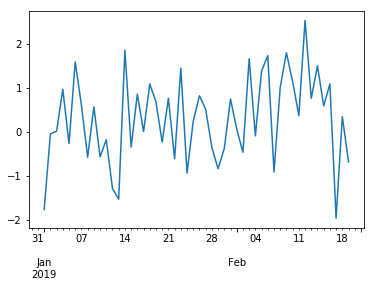In the above example, we call the plot method directly on pandas Series object ts. Alternatively, we could have called plt.plot(ts). Calling ts.plot() is equivalent to calling plt.plot(ts) and both calls would result in almost the same output as shown above. Additionally, the plot() method on pandas object supports almost every attribute that plt.plot() supports for formatting. For example, calling the plot method on pandas objects with a color attribute would result in a plot with color mentioned by its value. This is shown below:

""" Figure 16 """
# Plotting pandas Series in green color
ts.plot(color='green')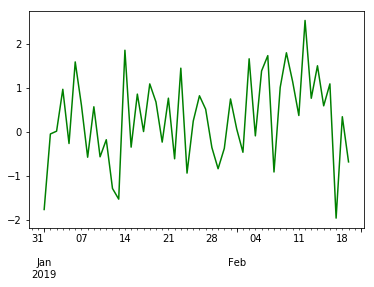Moving forward, the same notation is followed by pandas DataFrame object and visualizing data within a dataframe becomes more intuitive and less quirky. Before we attempt to plot data directly from a dataframe, let us create a new dataframe and populate it. We fetch the stock data of AAPL ticker that we will be using for illustration purposes throughout the remaining part of the Python matplotlib tutorial.

""" Script to fetch AAPL data from a web resource """
# Import libraries
import pandas as pd

# Fetch data


The dataframe data will contain stock data with dates being the index. The excerpt of the downloaded data is shown below:

DateOpenHighLowCloseVolumeExDividend...
2018-03-27173.68175.15166.92168.34038962893.00.0...
2018-03-26168.07173.10166.44172.77036272617.00.0...
2018-03-23168.39169.92164.94164.94040248954.00.0...

Now we can plot any column of a data dataframe by calling plot method on it. In the example given below, we plot the recent 100 data points from the Volume column of the dataframe:

""" Figure 17 """
# Plot volume column
data.Volume.iloc[:100].plot()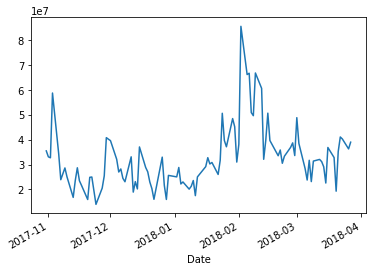With a dataframe, plot method is a convenience to plot all of the columns with labels. In other words, if we plot multiple columns, it would plot labels of each column as well.

""" Figure 18 """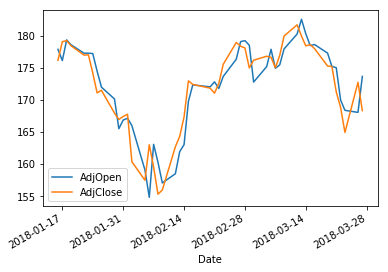The plot method generates a line plot by default when called on pandas data structures. However, it can also produce a variety of other charts as we will see later in this Python matplotlib tutorial. Having said that, let us head forward to plot scatter plots.

### Scatter Plot

In Python matplotlib, scatter plots are used to visualize the relationship between two different data sets. Python Matplotlib provides the scatter method within pyplot sub-module using which scatter plots can be generated.

• plt.scatter generates a scatter plot of y vs x with varying marker size and/or color.

The x and y parameters are data positions and it can be array-like sequential data structures. There are some instances where we have data in the format that lets us access particular variables with string. For example, Python dictionary or pandas dataframe. Python Matplotlib allows us to provide such an object with the data keyword argument to the scatter method to directly plot from it. The following example illustrates this using a dictionary.

""" Figure 19 """
# Creating a dictionary with three key-value pairs
dictionary = {'a': np.linspace(1, 100, 50),
'c': np.random.randint(0, 50, 50),
'd': np.abs(np.random.randn(50)) * 100}

# Creating a new dictionary key-value pair
dictionary['b'] = dictionary['a'] * np.random.rand(50)

# Plotting a scatter plot using argument 'data'
plt.scatter('a', 'b', c='c', s='d', data=dictionary)

# Labeling the plot and showing it
plt.xlabel('A Data Points')
plt.ylabel('B Data Points')
plt.show()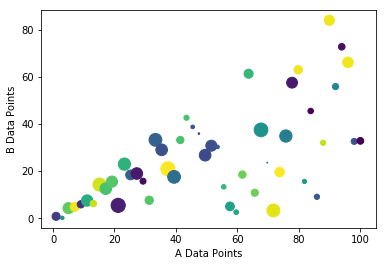In the above code, we created a dictionary dictionary with four key-value pairs. Values in key a and b contain fifty random values to be plotted on a scatter plot. Key c contains fifty random integers and key d contains fifty positive floats which represent color and size respectively for each scatter data point. Then, a call to plt.scatter is made along with all keys and the dictionary as the value to data. The argument c within the call refers to color to be used and the argument s represents the size of a data point. These arguments c and s are optional. A simple scatter plot with the same color and size gets plotted when we omit these optional arguments as shown in the following example:

""" Figure 20 """
# Creating a scatter plot without color and same
plt.scatter(dictionary['a'], dictionary['b'])

# Labeling the plot and showing it
plt.xlabel('A Data Points')
plt.ylabel('B Data Points')
plt.show()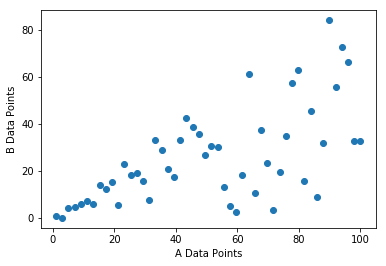To better understand the working of scatter plots, let us resort to our old friends: lists x and y that we defined earlier while learning line plots and build scatter plots on them. To refresh our memory, we re-define the same lists below:

# Data points for scatter plot
x = [1.3, 2.9, 3.1, 4.7, 5.6, 6.5, 7.4, 8.8, 9.2, 10]
y = [95, 42, 69, 11, 49, 32, 74, 62, 25, 32]


In addition to these lists, we would be defining two more NumPy arrays color and size which determines the color and size respectively of each data point while plotting the scatter plot.

# Arrays which defines color and size of each data point
color = np.random.rand(10)
size = np.random.randint(50, 100, 10)


Now that we have data points ready, we can plot a scatter plot out of them as below:

""" Figure 21 """
# Creating a scatter plot
plt.scatter(x, y, c=color, s=size)

# Labeling the plot and showing it
plt.xlabel('Values from list x')
plt.ylabel('Values from list y')
plt.show()


The scatter plot would contain data points each with different color and size (as they are randomly generated). The output is shown below: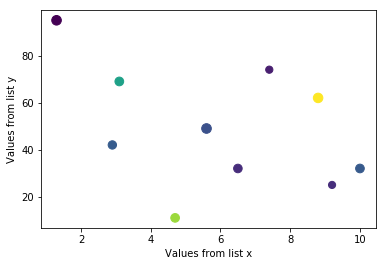In the financial domain, scatter plots are widely used to determine the relations between two data sets visually. That being said and being equipped with the working knowledge of scatter plot, let us now move forward in the Python matplotlib tutorial and plot a scatter plot of AdjOpen and AdjClose prices of AAPL stock that we have in pandas dataframe data. When it comes to plotting data directly from a pandas dataframe, we can almost always resort to plot method on pandas to plot all sorts of plots. That is, we can directly use the plot method on the dataframe to plot scatter plots even just like line plots. However, we need to specify that we are interested in plotting a scatter plot using the argument kind='scatter' as shown below:

""" Figure 22 """
# Plotting a scatter plot of 'AdjOpen' and 'AdjClose' of AAPL stock
plt.show()


Interestingly, we only need to specify column names of a dataframe data for x and y coordinates along with the argument kind which gets resulted in the output as shown below: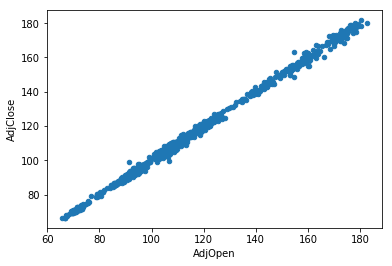By visualizing price patterns using a scatter plot, it can be inferred that open and close prices are positively correlated. Furthermore, we can generate the same plot using the plt.scatter method.

""" Method 1 """
plt.show()

""" Method 2 """
plt.show()


The method one uses the argument data which specifies the data source, whereas the second method directly uses dataframe slicing and hence, there is no need to specify the data argument.

### Histogram Plots

A histogram is a graphical representation of the distribution of data. It is a kind of bar graph and a great tool to visualize the frequency distribution of data that is easily understood by almost any audience. To construct a histogram, the first step is to bin the range of data values, divide the entire range into a series of intervals and finally count how many values fall into each interval. Here, the bins are consecutive and non-overlapping. In other words, histograms show the data in the form of some groups. All the bins/groups go on X-axis, and Y-axis shows the frequency of each bin/group.

The Python matplotlib library offers a very convenient way to plot histograms. To create a histogram, we use the hist method of pyplot sub-module of the matplotlib library. Let us start by creating a simple histogram.

""" Figure 23 """
# Data values for creating a histogram
y = [95, 42, 69, 11, 49, 32, 74, 62, 25, 32]

# Creating a histogram
plt.hist(y)
plt.xlabel('Bins')
plt.ylabel('Frequency')
plt.show()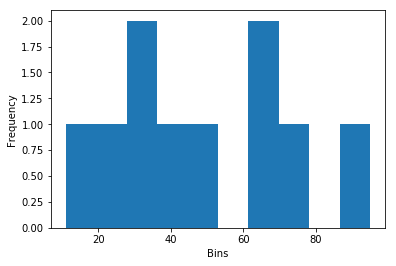In Python matplotlib, this is the simplest code possible to plot a histogram with minimal arguments. We create a range of values and simply provide it to the hist method and let it perform the rest of the things (creating bins, segregating each value to the corresponding bin, plotting, etc.). The hist method also takes bins as an optional argument. If this argument is specified, bins will be created as per the specified value, otherwise, it will create bins on its own. To illustrate this, we explicitly specify the number of bins in the above code and generate the plot. The modified code and output is shown below:

""" Figure 24 """
# Data values for creating a histogram
y = [95, 42, 69, 11, 49, 32, 74, 62, 25, 32]

# Creating a histogram
plt.hist(y, bins= 20)
plt.xlabel('Bins')
plt.ylabel('Frequency')
plt.show()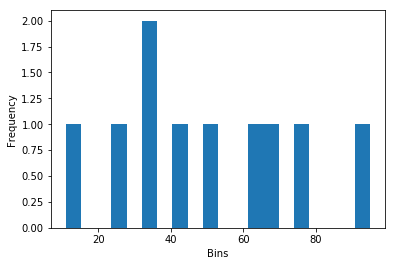The output we got is very straight forward. Number 32 appears twice in the list y which reflects intuitively. We specify the number of bins to be 20 and hence, the hist method tries to divide the whole range of values into 20 bins and plots them on the X-axis. Similar to the plot method, the hist method also takes any sequential data structure as its input and plots histogram of it. Let us try to generate a histogram of an array in Python matplotlib which draws samples from the standard normal distribution.

""" Figure 25 """
# Creating an array
array = np.random.normal(0, 1, 10000)

# Creating a histogram
plt.hist(array)
plt.xlabel('Bins')
plt.ylabel('Frequency')
plt.show()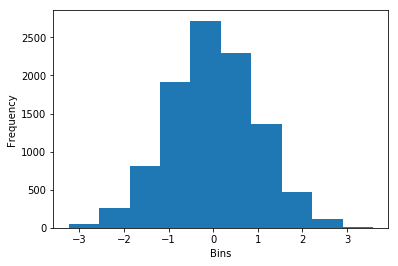The output we got shows that the data distribution indeed resembles a normal distribution. Apart from bins argument, other arguments that can be provided to hist are color and histtype. There are lot many arguments that can be provided, but we will keep our discussion limited to these few arguments only. The color of a histogram can be changed using the color argument. The histtype argument takes some of the pre-defined values such as bar, barstacked, step and stepfilled. The below example illustrates the usage of these arguments.

""" Figure 26 """
# Creating an array
array = np.random.normal(0, 1, 10000)

# Creating a histogram and plotting it
plt.hist(array, color='purple', histtype='step')
plt.xlabel('Bins')
plt.ylabel('Frequency')
plt.show()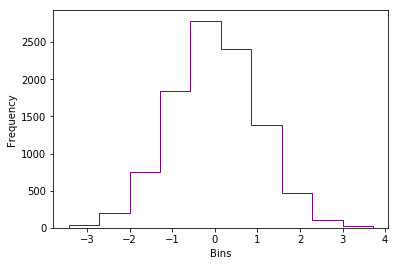In addition to optional arguments discussed so far, one argument that needs attention is orientation. This argument takes either of two values: horizontal or vertical. The default is vertical.

""" Figure 27 """
# Creating an array
array = np.random.normal(0, 1, 10000)

# Creating a histogram and plotting it
plt.hist(array, color='teal', orientation='horizontal')
plt.xlabel('Frequency')
plt.ylabel('Bins')
plt.show()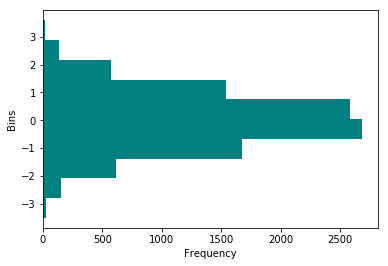We now shift our focus on plotting a histogram directly from a pandas dataframe in Python matplotlib. Again, the plot method within pandas provides a wrapper around the hist function in Python matplotlib as it was the case with scatter plots. To plot a histogram, we need to specify the argument kind with the value hist when a call to plot is made directly from the dataframe. We will be working with the same dataframe data that contains historical data for AAPL stock.

""" Figure 28 """
# Method 1
# Creating a histogram using a dataframe method
data['Volume'].plot(kind='hist')
plt.show()

# Method 2
plt.hist(data['Volume'])
plt.ylabel('Frequency')
plt.show()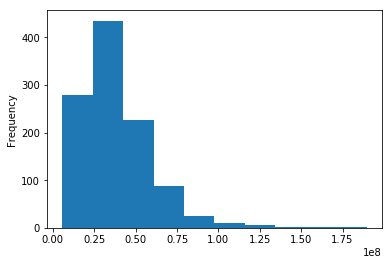In the first method, we directly make a call to plot method on the dataframe data sliced with Volume column. Whereas in the second method, we use the hist method provided by matplotlib.pyplot module to plot the histogram. Both methods plot the same result as shown above. In the next section of the Python matplotlib tutorial, we are going to learn something interesting, how to customise your own plots.

## Plot Customization using Python Matplotlib

Now that we have got a good understanding of plotting various types of charts and their basic formatting techniques, we can delve deeper and look at some more formatting techniques. We already learned that Python matplotlib does not add any styling components on its own. It will plot a simple plain chart by default. We as a user need to specify whatever customization we need. We start with a simple line plot and will keep on making it better. The following example shows plotting of close prices of the AAPL ticker that is available with us in the dataframe data.

""" Figure 29 """
# Extracting close prices from the dataframe

# Plotting the close prices
plt.plot(close_prices)
plt.show()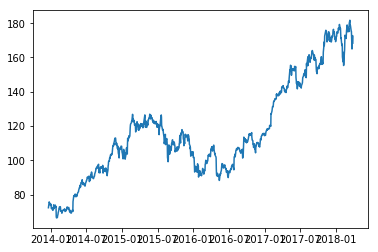Here, the close_prices is the pandas Series object which gets plotted using the plot method. However, values on the X-axis are something that we don't want. They are all overlapped with each other. This happens as the plot method did not find sufficient space for each date. One way to overcome this issue is to rotate the values on the X-axis to make it look better.

""" Figure 30 """
plt.plot(close_prices)

# Rotating the values along x-axis to 45 degrees
plt.xticks(rotation=45)
plt.show()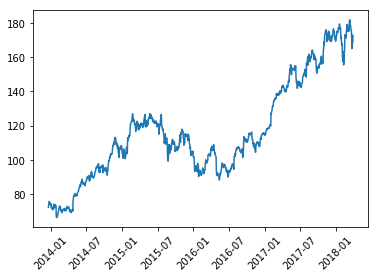The xticks method along with the rotation argument is used to rotate the values/tick names along the x-axis. Another approach that can be used to resolve the overlapping issue is to increase the figure size of the plot such that the Python matplotlib can easily show values without overlapping. This is shown in the below example:

""" Figure 31 """
# Creating a figure with the size 10 inches by 5 inches
fig = plt.figure(figsize=(10, 5))
plt.plot(close_prices)
plt.show()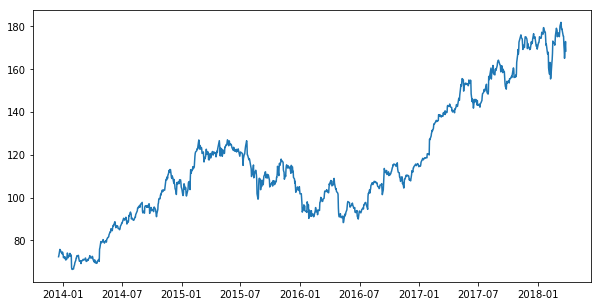Similarly, Python matplotlib provides yticks method that can be used to customize the values on the Y-axis. Apart from the rotation argument, there are a bunch of other parameters that can be provided xticks and yticks to customize them further. We change the font size, color and orientation of ticks along the axes using the appropriate arguments within these methods in the following example:

""" Figure 32 """
# Creating a figure, setting its size and plotting close prices on it
fig = plt.figure(figsize=(10, 5))
plt.plot(close_prices, color='purple')

# Customizing the axes
plt.xticks(rotation=45, color='teal', size=12)
plt.yticks(rotation=45, color='teal', size=12)

# Setting axes labels
plt.xlabel('Dates', {'color': 'orange', 'fontsize':15})
plt.ylabel('Prices', {'color': 'orange', 'fontsize':15})
plt.show()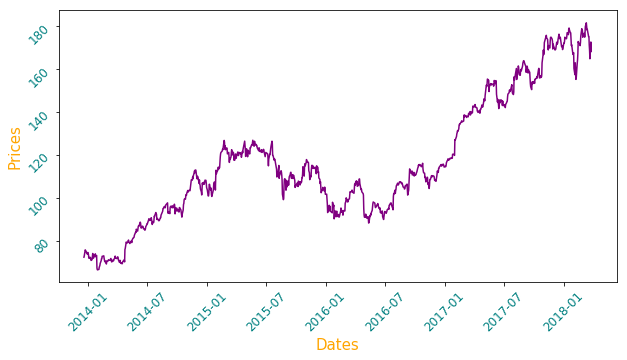Along with the axes values, we change the color and font size of axes labels. There are numbers of other customization possible using various arguments and Python matplotlib provides total flexibility to create the charts as per one's desire. Two main components that are missing in the above plot are title and legend, which can be provided using the methods title and legends respectively. Again, as with the other methods, it is possible to customize them in a variety of way, but we will be restricting our discussing to few important arguments only. Adding these two methods as shown below in the above code would produce the following plot:

""" Figure 33 """
# Showing the legend and setting the title of plot
plt.legend()
plt.title('AAPL Close Prices', color='purple', size=20)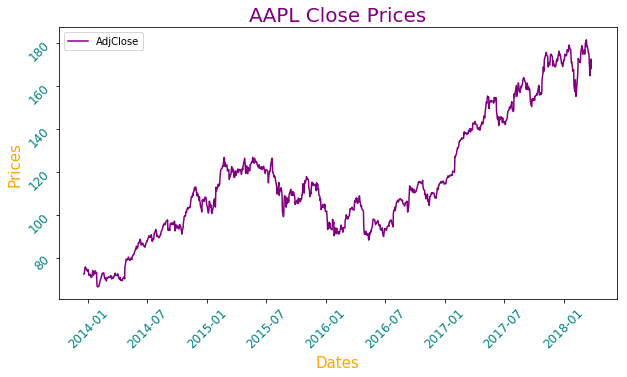Another important feature in Python matplotlib that can be added to a figure is to draw a grid within a plot using the grid method which takes either True or False. If true, a grid is plotted, otherwise not.

""" Figure 34 """
# Adding the grid to the plot
plt.grid(True)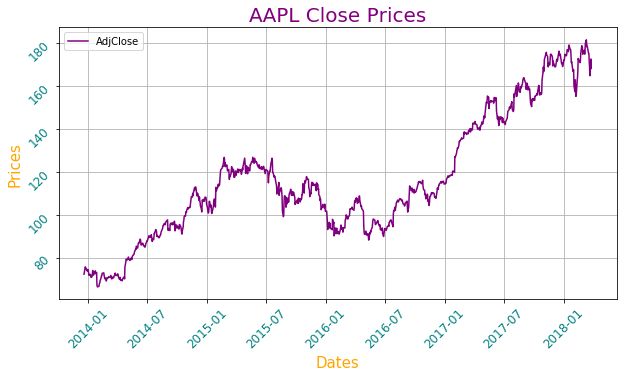The axhline method allows us to add a horizontal line across the axis to the plot. For example, we might consider adding the mean value of close prices to show the average price of a stock for the whole duration. It can be added using axhline method in Python matplotlib. Computation of mean value and its addition to the original plot is shown below:

""" Figure 35 """
# Importing NumPy library
import numpy as np

# Calculating the mean value of close prices
mean_price = np.mean(close_prices)

# Plotting the horizontal line along with the close prices
plt.axhline(mean_price, color='r', linestyle='dashed')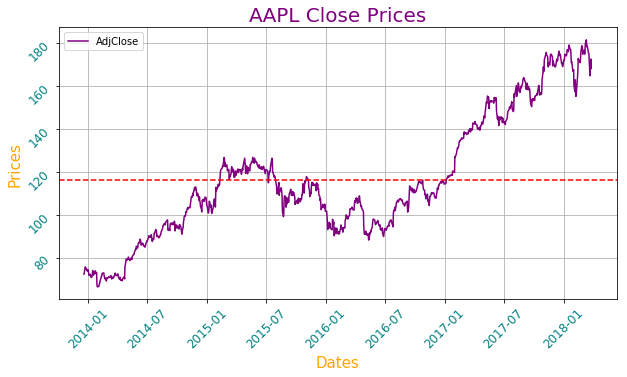Now that we have the mean value of close prices plotted in the figure, one who looks at the chart for the first time might think what this red line conveys? Hence, there is a need to explicitly mention it. To do so, we can use the text method provided by matplotlib.pyplot module to plot text anywhere on the figure.

""" Figure 36 """
# Importing DateTime from DateTime library
from datetime import datetime

# Plotting text on date 2014-1-7 and price 120
plt.text(datetime(2014,1,7), 120, 'Mean Price', size=15, color='r')


The text method takes three compulsory arguments: x, y and t which specifies the coordinates on X and Y-axis and text respectively. Also, we use a datetime sub-module from a datetime library to specify a date on the X-axis as the plot we are generating has dates on the X-axis. The chart with text indicating the mean price is shown below: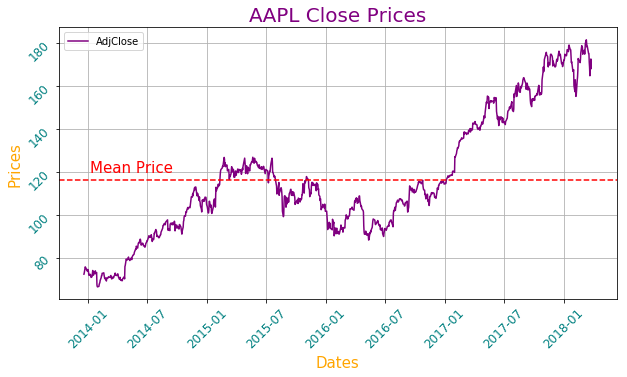Using all these customization techniques, we have been able to evolve the dull-looking price series chart to a nice and attractive graphic which is not only easy to understand but presentable also. However, we have restricted ourselves to plotting only a single chart. Let us brace ourselves and learn to apply these newly acquired customization techniques to multiple plots.

## Multiple Plots using Python Matplotlib

We already learned at the beginning of this Python matplotlib tutorial that a figure can have multiple plots, and that can be achieved using the subplots method. The following examples show stock prices of AAPL stock along with its traded volume on each day.

""" Figure 37 """

# Extracting volume from the dataframe 'data'

# Creating figure with two rows and one column
fig, (ax1, ax2) = plt.subplots(nrows=2, ncols=1, sharex=True, figsize=(10, 8))

# Plotting close prices on the first sub-plot
ax1.plot(close_prices, color='purple')
ax1.grid(True)

# Plotting trading volume on the second sub-plot
ax2.bar(volume.index, volume)
ax2.grid(True)

# Displaying the plot
plt.show()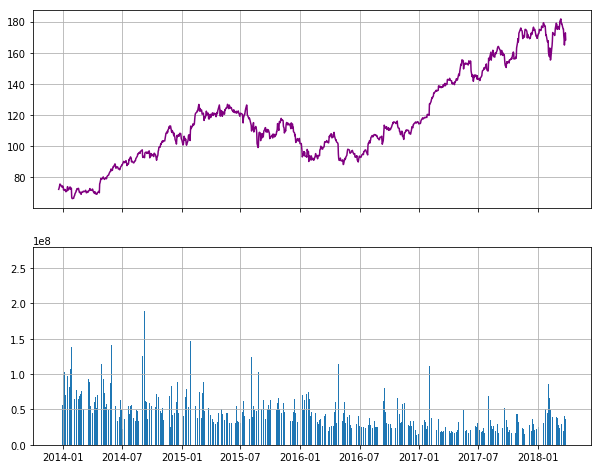First, we extract the AdjVolume column from the data dataframe into a volume which happens to be pandas series object. Then, we create a figure with sub-plots having two rows and a single column. This is achieved using nrows and ncols arguments respectively. The sharex argument specifies that both sub-plots will share the same x-axis. Likewise, we also specify the figure size using the figsize argument. These two subplots are unpacked into two axes: ax1 and ax2 respectively. Once, we have the axes, desired charts can be plotted on them.

Next, we plot the close_prices using the plot method and specify its color to be purple using the color argument. Similar to the plot method, Python matplotlib provides bar method to draw bar plots which takes two arguments: the first argument to be plotted on the X-axis and second argument to be plotted along the y-axis. For our example, values on X-axis happens to be a date (specified by volume.index), and value for each bar on the Y-axis is provided using the recently created volume series. After that, we plot grids on both plots. Finally, we display both plots. As can be seen above, Python matplotlib rendered a decent chart. However, it misses some key components such as title, legends, etc.

""" Figure 38 """
# Creating figure with multiple plots
fig, (ax1, ax2) = plt.subplots(nrows=2, ncols=1, sharex=True, figsize=(10, 8))
ax1.plot(close_prices, color='purple', label='Prices')
ax1.grid(True)

# Setting the title of a first plot
ax1.set_title('Daily Prices')

# Setting the legend for the first plot
ax1.legend()

ax2.bar(volume.index, volume, label='Volume')
ax2.grid(True)

# Setting the title of a second plot
ax2.set_title('Volume')

# Setting the legend for the second plot
ax2.legend()

plt.show()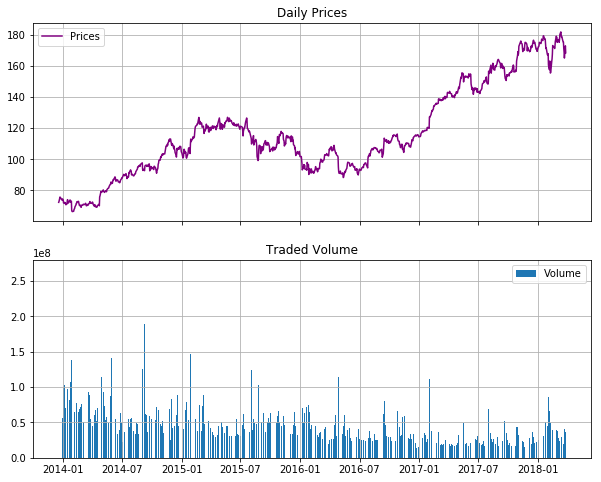Here, we use the legend method to set legends in both plots. Legends will print the values specified by the label argument while plotting each plot. The set_title is used to set the title for each plot. Earlier, while dealing with a single plot, we had used the title method to set the title. However, it doesn't work the same way with multiple plots.

Another handy method provided by Python matplotlib is the tight_layout method which automatically adjusts the padding and other similar parameters between subplots so that they fit into the figure area.

""" Figure 39 """
# Setting layout
plt.tight_layout()

# Setting label on the x-axis
plt.xlabel('Dates')
plt.show()


The above code explicitly specifies the layout and the label on the x-axis which results in the following chart.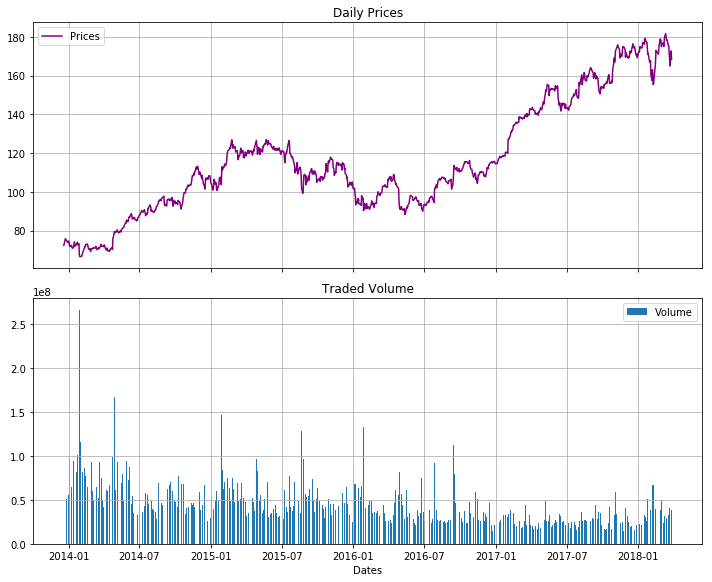In addition to all this customization, Python matplotlib also provides a number of predefined styles that can be readily used. For example, there is a predefined style called “ggplot”, which emulates the aesthetics of ggplot (a popular plotting package for R language). To change the style of plots being rendered, the new style needs to be explicitly specified using the following code:

plt.style.use('ggplot')


One the style is set to use, all plots rendered after that will use the same and newly set style. To list all available styles, execute the following code:

plt.style.available


Let us set the style to one of the pre-defined style known as 'fivethirtyeight' and plot the chart.

plt.style.use('fivethirtyeight')
fig, (ax1, ax2) = plt.subplots(nrows=2, ncols=1, sharex=True, figsize=(10, 8))

ax1.plot(close_prices, color='purple', label='Prices')
ax1.grid(True)
ax1.set_title('Daily Prices')
ax1.legend()

ax2.bar(volume.index, volume, label='Volume')
ax2.grid(True)
ax2.legend()

plt.tight_layout()

plt.xlabel('Dates')
plt.show()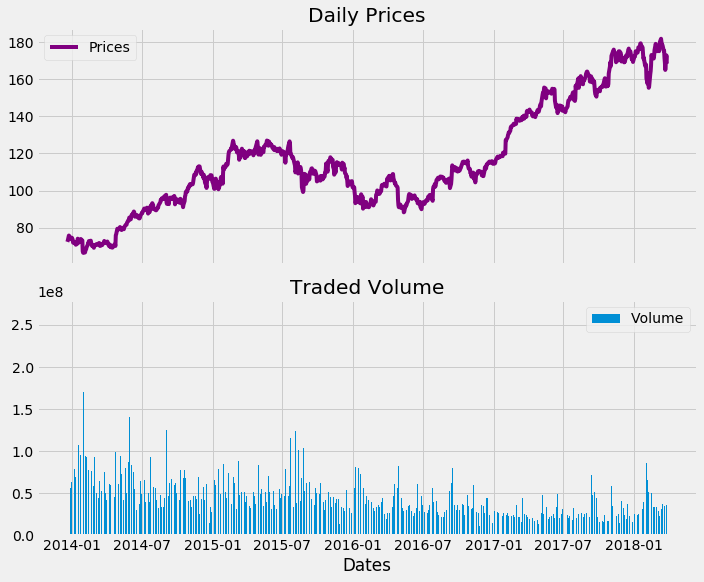By changing the style, we get a fair idea about how styles play an important role to change the look of charts cosmetically while plotting them.

The last method that we will study is the savefig method that is used to save the figure on a local machine. It takes the name of the figure by which it will be saved. This is illustrated below:

plt.savefig('AAPL_chart.png')


Executing the above code will save the chart we plotted above with the name AAPL_chart.png.

Thus, in this Python matplotlib tutorial, we started with the basics of figure and plots, gradually learning various types of charts and their nitty-gritty along the way, and finally, we learned customization and took a sneak-peek into plotting multiple plots within the same chart.

To reiterate, the Python matplotlib tutorial is an excerpt from the Python Basics Handbook, which was created for both; beginners who are starting out in Python as well as accomplished traders who can use it as a handy reference while coding their strategy.

Do let us know if you loved the article and any other feedback in the comments below.

Disclaimer: All investments and trading in the stock market involve risk. Any decisions to place trades in the financial markets, including trading in stock or options or other financial instruments is a personal decision that should only be made after thorough research, including a personal risk and financial assessment and the engagement of professional assistance to the extent you believe necessary. The trading strategies or related information mentioned in this article is for informational purposes only.